# Alluvial plot in ggplot2 with ggalluvial

Cory Brunson

## Sample data set

The `vaccinations` data set from the `ggalluvial` package contains a survey “that asked respondents their probability of vaccinating for influenza”. The `response` variable is the discretized probability of vaccinating, `freq` the number of respondents and `survey` the survey designation.

``````# install.packages("ggalluvial")
library(ggalluvial)

vaccinations``````

## Alluvial plot

The `ggalluvial` package provides the `geom_alluvium` and `geom_stratum` functions to create alluvial diagrams in ggplot2. You will need to pass your data in long format, specify the axis variables inside `aes` and use the previous functions.

Note that you can also add the text for each stratum, add the names of the axis variables (in the following examples you won’t see them because we set `theme_void`) and change the ggplot2 theme, among other customizations.

``````# install.packages("ggalluvial")
library(ggalluvial)

ggplot(data = vaccinations,
aes(axis1 = survey, axis2 = response, y = freq)) +
geom_alluvium(aes(fill = response)) +
geom_stratum() +
geom_text(stat = "stratum",
aes(label = after_stat(stratum))) +
scale_x_discrete(limits = c("Survey", "Response"),
expand = c(0.15, 0.05)) +
theme_void()``````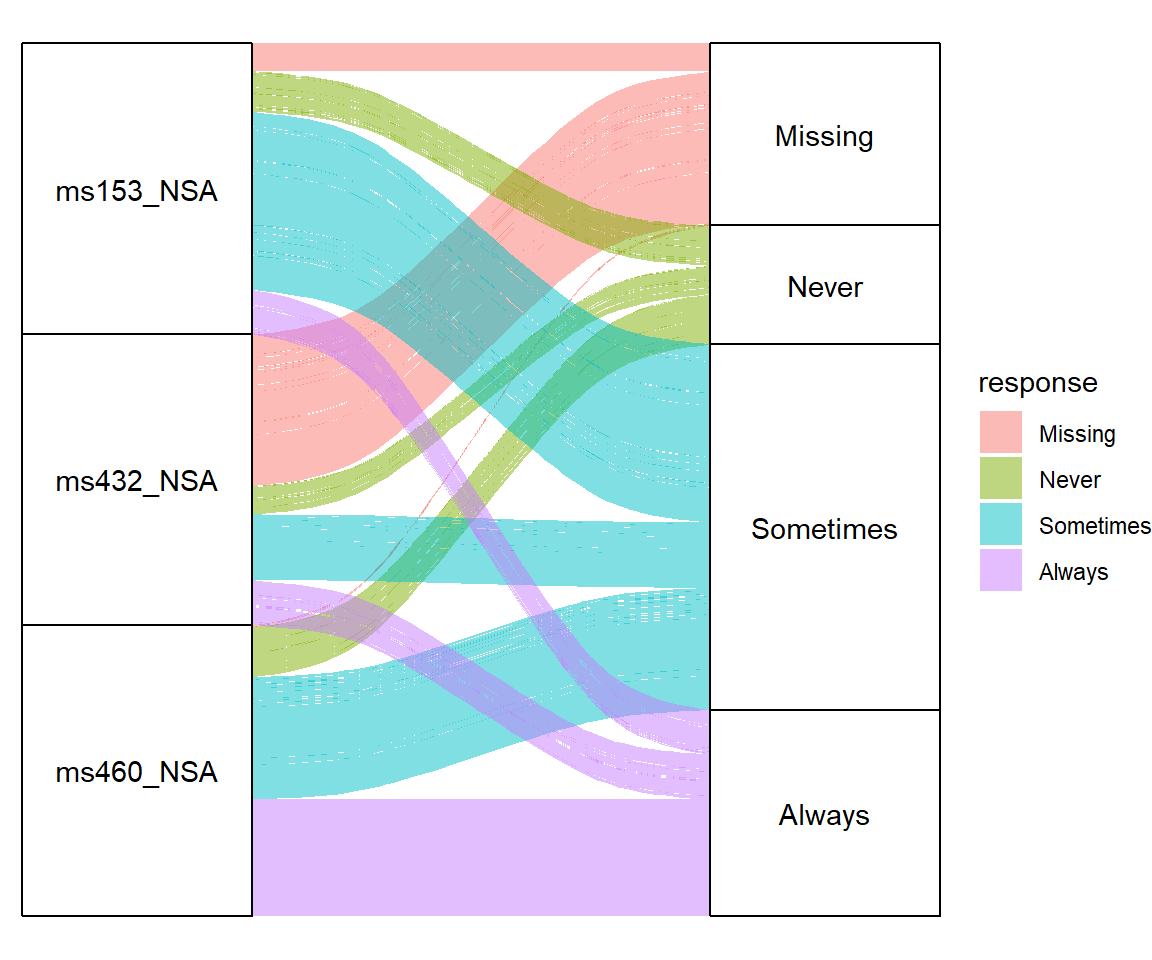If your data set contains more categorical variables you can pass them to `aes` (`axis1`, `axis2`, `axis3` …) as shown in the example below.

``````# install.packages("ggalluvial")
library(ggalluvial)

ggplot(data = vaccinations,
aes(axis1 = survey,   # First variable on the X-axis
axis2 = response, # Second variable on the X-axis
axis3 = survey,   # Third variable on the X-axis
y = freq)) +
geom_alluvium(aes(fill = response)) +
geom_stratum() +
geom_text(stat = "stratum",
aes(label = after_stat(stratum))) +
scale_x_discrete(limits = c("Survey", "Response"),
expand = c(0.15, 0.05)) +
theme_void()``````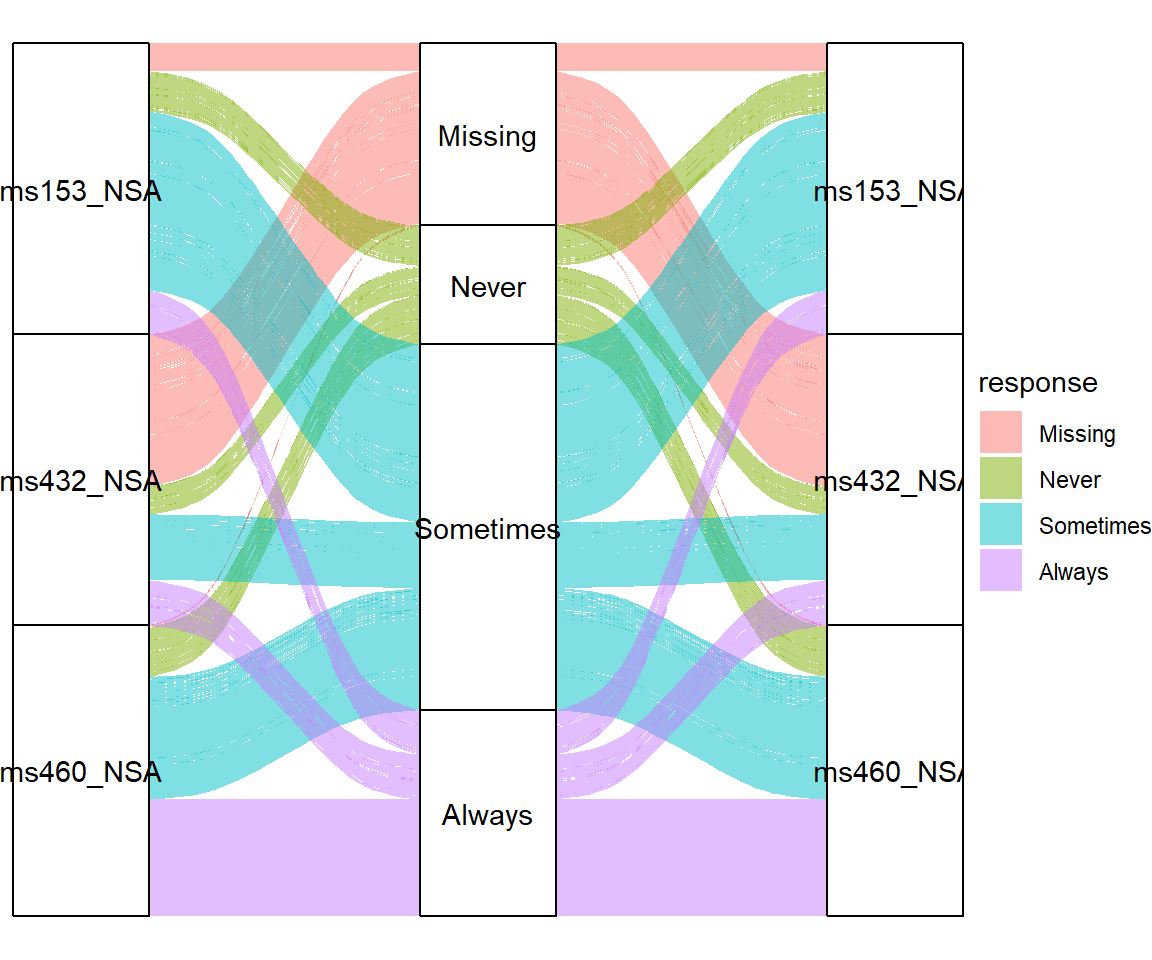## Curve types

The type of flows of the plot area can be customized with the `curve_type` argument of the `geom_alluvium` function. The default value is `"xspline"`, which produces approximation splines using four points per curve. The alternatives are listed below: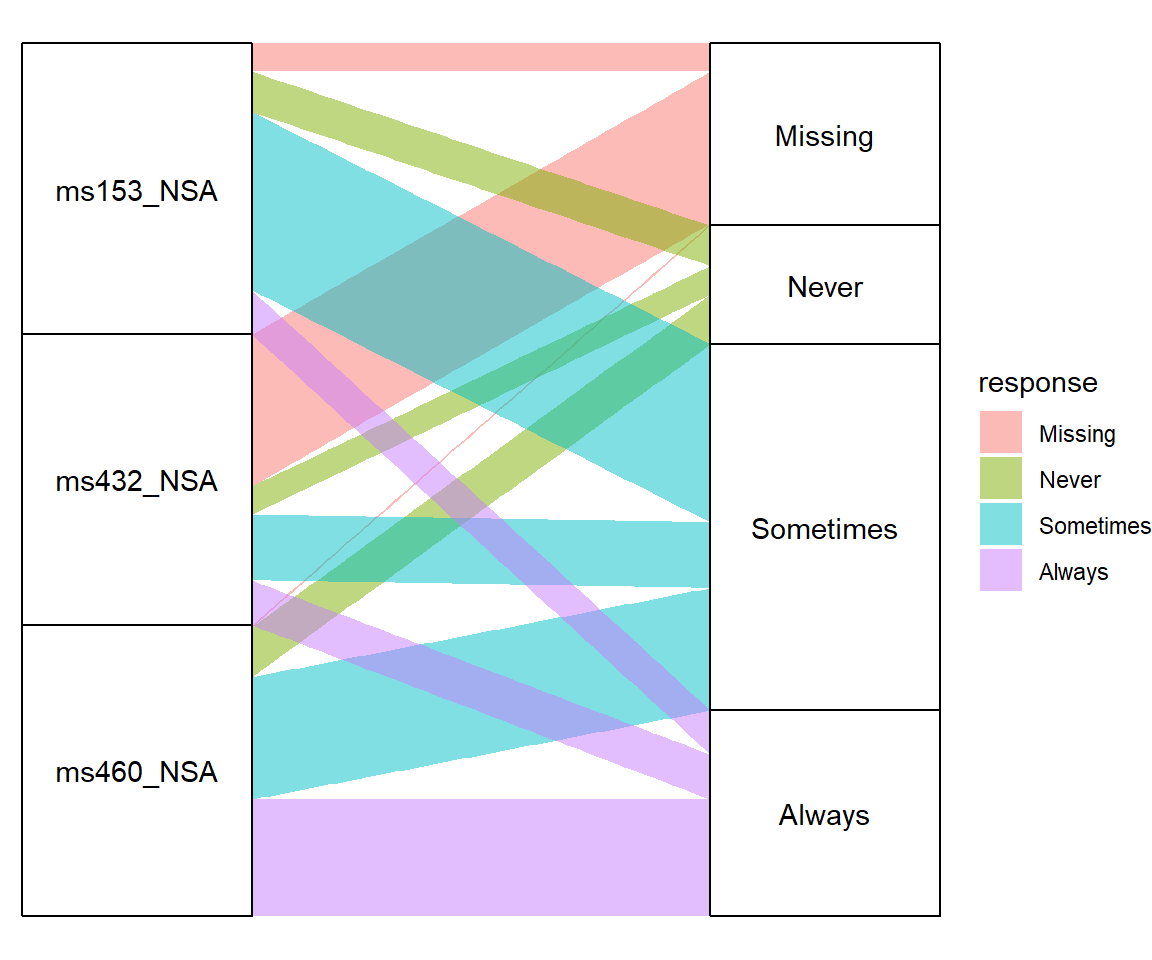Linear

``````# install.packages("ggalluvial")
library(ggalluvial)

ggplot(data = vaccinations,
aes(axis1 = survey, axis2 = response, y = freq)) +
geom_alluvium(aes(fill = response),
curve_type = "linear") +
geom_stratum() +
geom_text(stat = "stratum",
aes(label = after_stat(stratum))) +
scale_x_discrete(limits = c("Survey", "Response"),
expand = c(0.15, 0.05)) +
theme_void()``````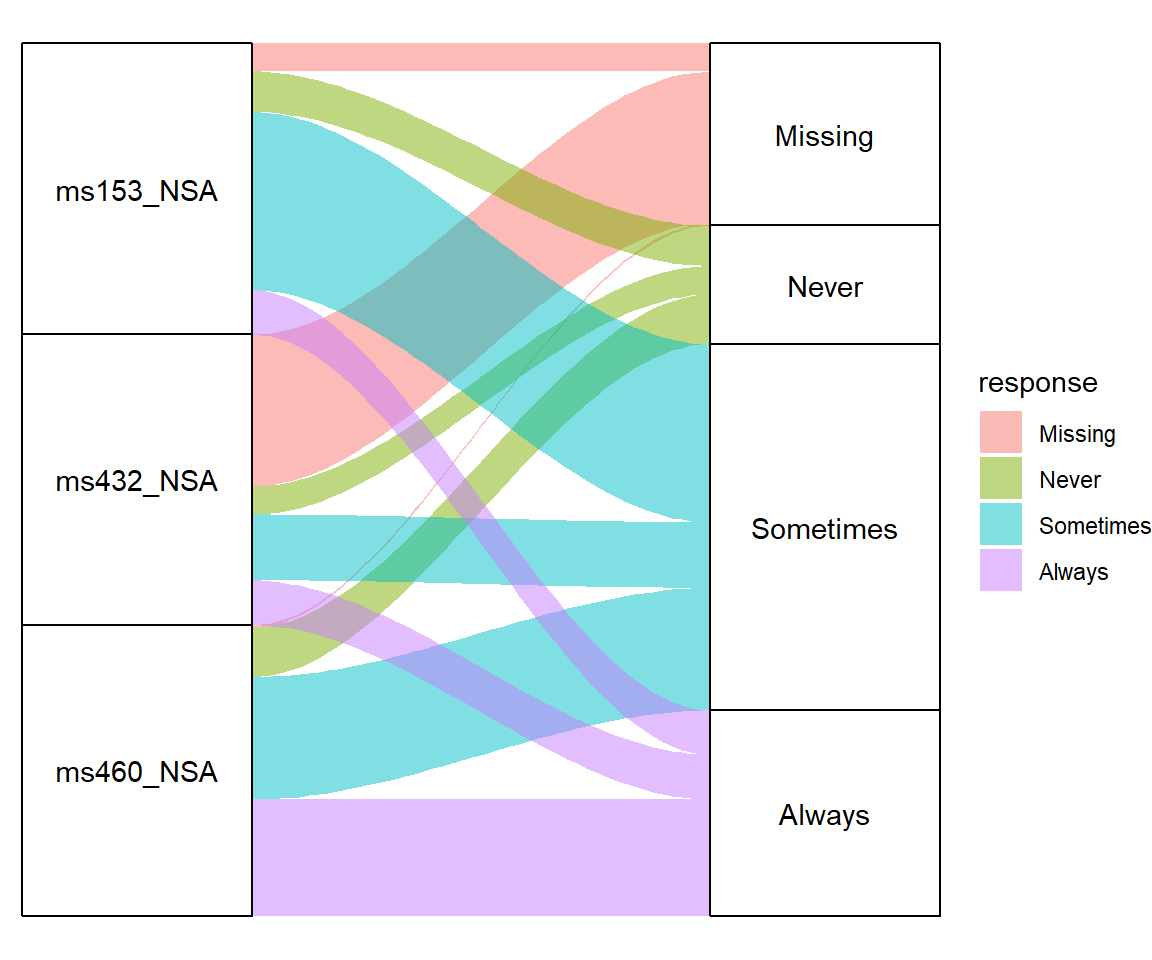Cubic

``````# install.packages("ggalluvial")
library(ggalluvial)

ggplot(data = vaccinations,
aes(axis1 = survey, axis2 = response, y = freq)) +
geom_alluvium(aes(fill = response),
curve_type = "cubic") +
geom_stratum() +
geom_text(stat = "stratum",
aes(label = after_stat(stratum))) +
scale_x_discrete(limits = c("Survey", "Response"),
expand = c(0.15, 0.05)) +
theme_void()``````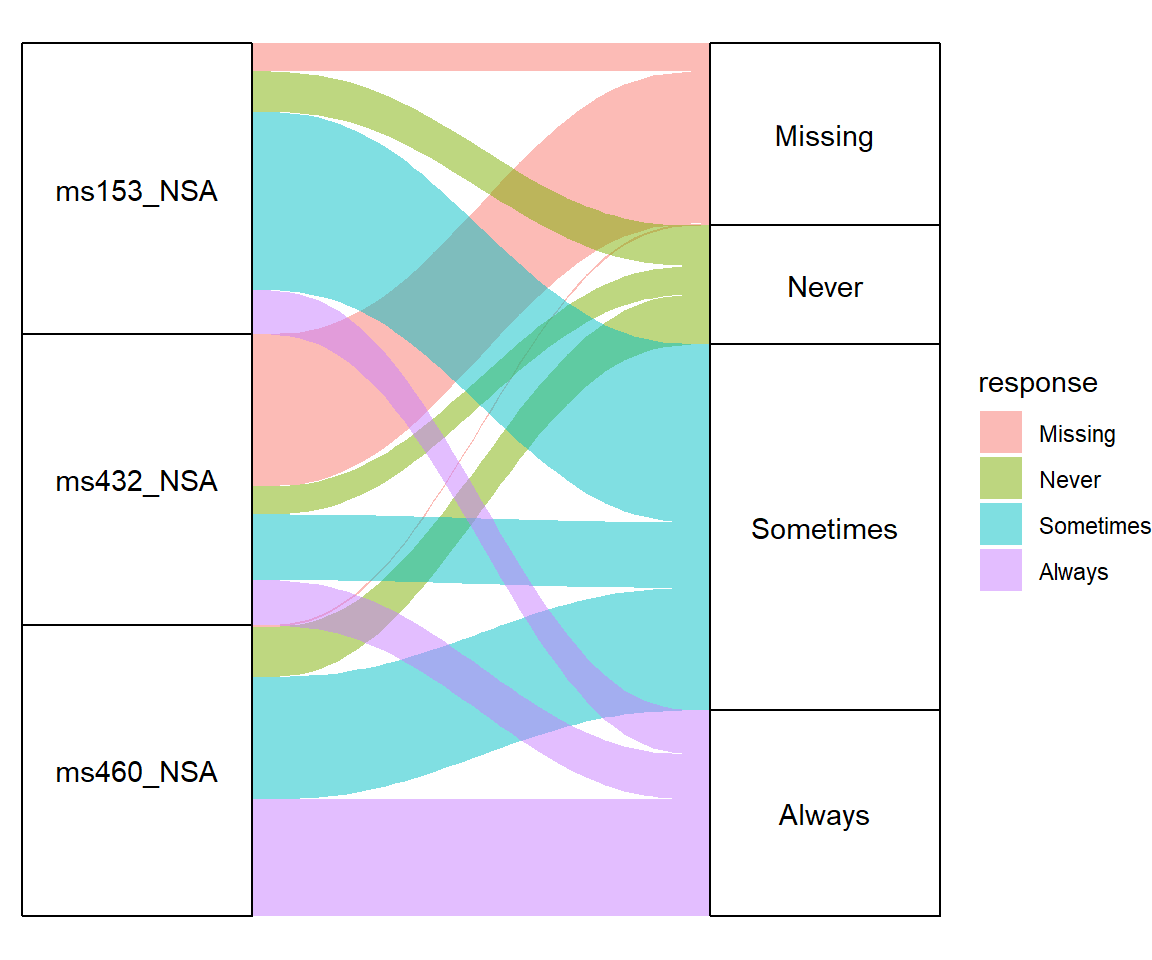Quintic

``````# install.packages("ggalluvial")
library(ggalluvial)

ggplot(data = vaccinations,
aes(axis1 = survey, axis2 = response, y = freq)) +
geom_alluvium(aes(fill = response),
curve_type = "quintic") +
geom_stratum() +
geom_text(stat = "stratum",
aes(label = after_stat(stratum))) +
scale_x_discrete(limits = c("Survey", "Response"),
expand = c(0.15, 0.05)) +
theme_void()``````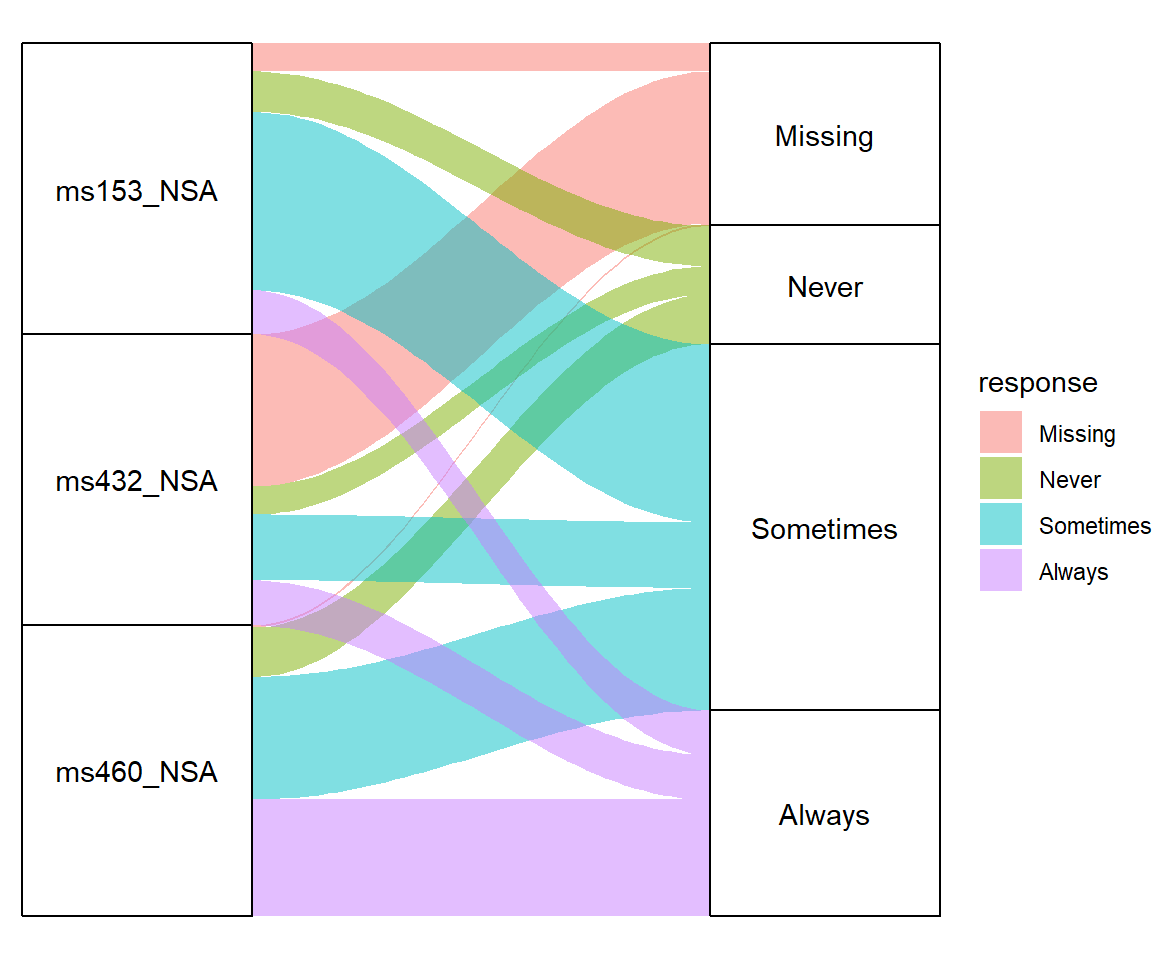Sine

``````# install.packages("ggalluvial")
library(ggalluvial)

ggplot(data = vaccinations,
aes(axis1 = survey, axis2 = response, y = freq)) +
geom_alluvium(aes(fill = response),
curve_type = "sine") +
geom_stratum() +
geom_text(stat = "stratum",
aes(label = after_stat(stratum))) +
scale_x_discrete(limits = c("Survey", "Response"),
expand = c(0.15, 0.05)) +
theme_void()``````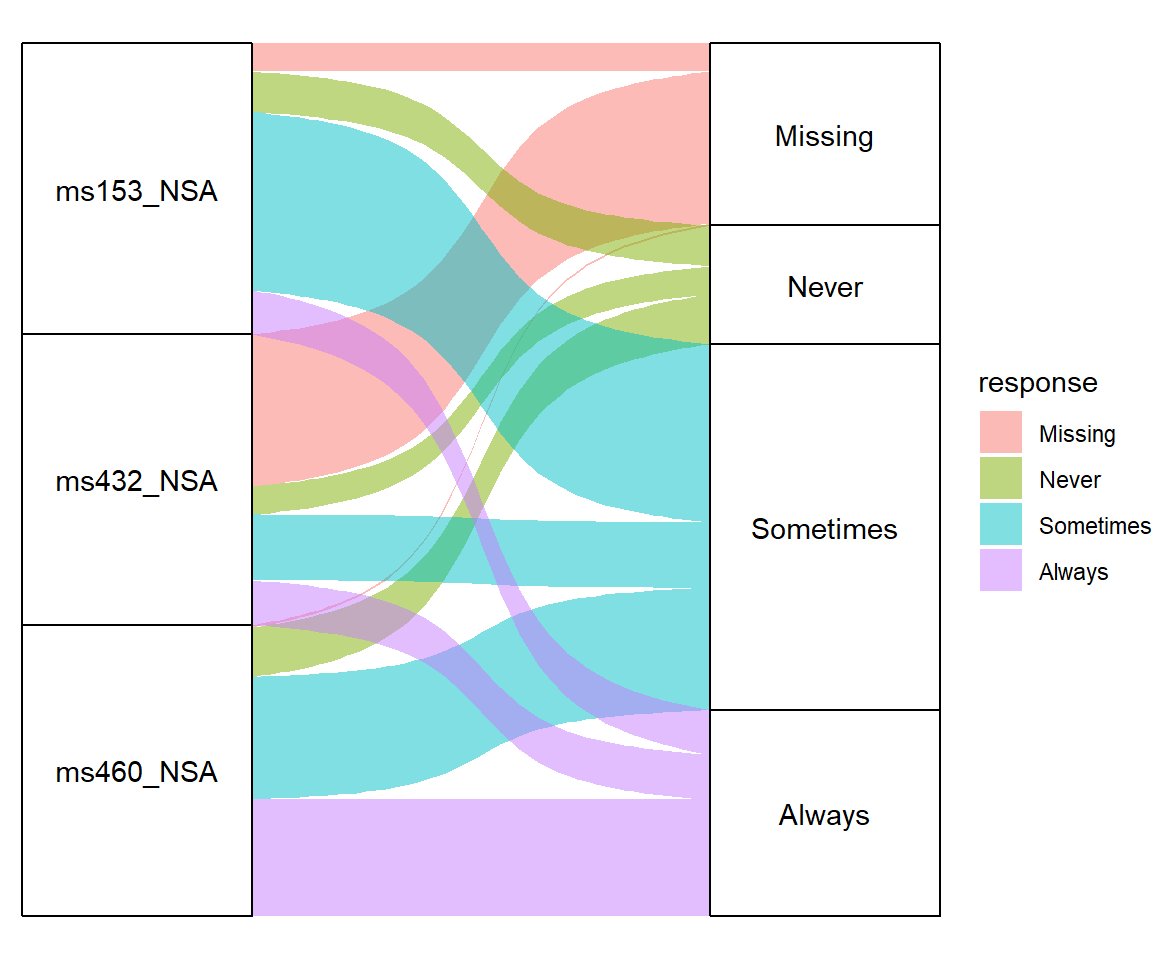Arctangent

``````# install.packages("ggalluvial")
library(ggalluvial)

ggplot(data = vaccinations,
aes(axis1 = survey, axis2 = response, y = freq)) +
geom_alluvium(aes(fill = response),
curve_type = "arctangent") +
geom_stratum() +
geom_text(stat = "stratum",
aes(label = after_stat(stratum))) +
scale_x_discrete(limits = c("Survey", "Response"),
expand = c(0.15, 0.05)) +
theme_void()``````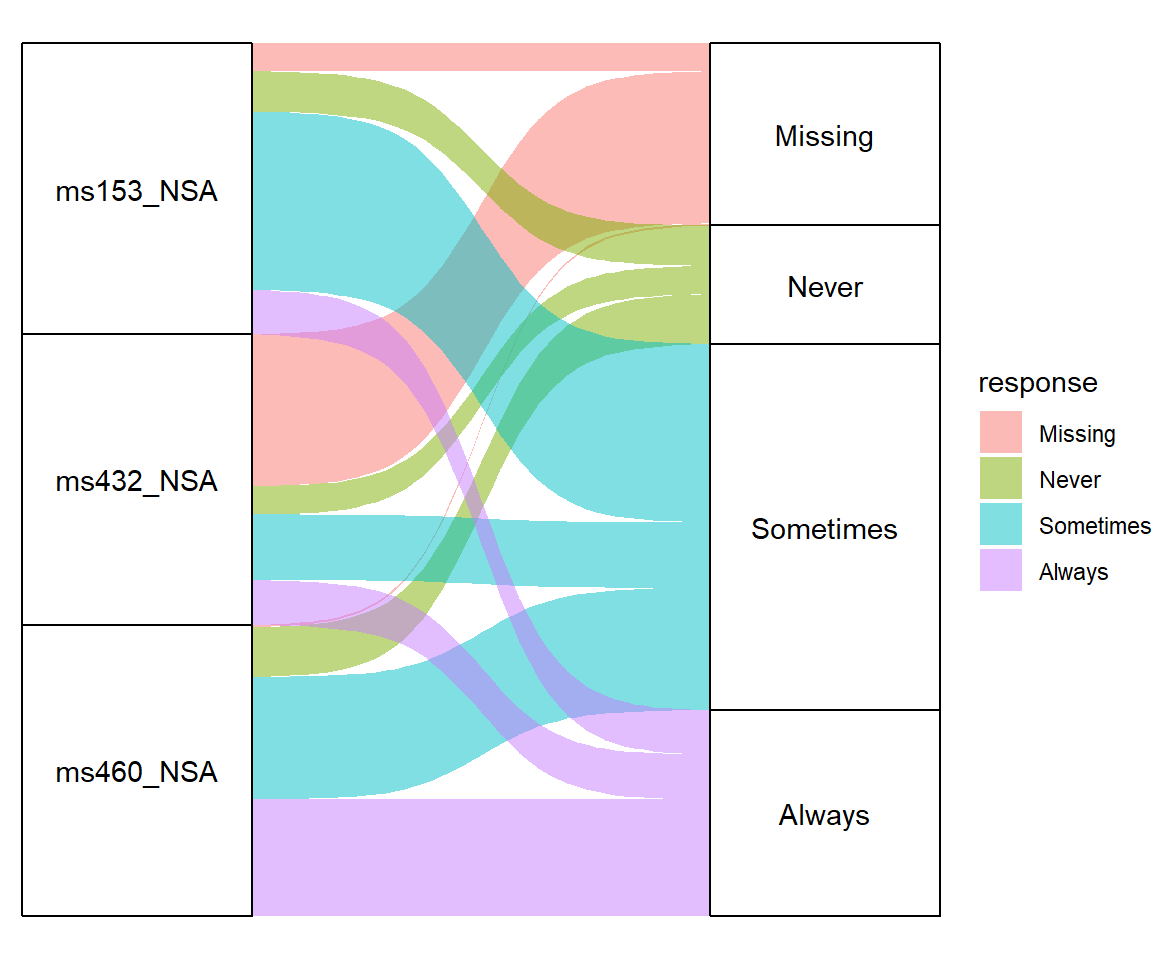Sigmoid

``````# install.packages("ggalluvial")
library(ggalluvial)

ggplot(data = vaccinations,
aes(axis1 = survey, axis2 = response, y = freq)) +
geom_alluvium(aes(fill = response),
curve_type = "sigmoid") +
geom_stratum() +
geom_text(stat = "stratum",
aes(label = after_stat(stratum))) +
scale_x_discrete(limits = c("Survey", "Response"),
expand = c(0.15, 0.05)) +
theme_void()``````

Note that there is an additional argument named `curve_range` for alternative curve types based on asymptotic functions. Type `geom_alluvium` for additional details.

## Color customization

It is possible to customize the colors of the flows. You can set a single color for them, use a predefined color palette or use your own vector of colors. Note that you can also modify the colors of the stratum boxes.

Fill color

``````# install.packages("ggalluvial")
library(ggalluvial)

ggplot(data = vaccinations,
aes(axis1 = survey, axis2 = response, y = freq)) +
geom_alluvium(aes(fill = "red")) +
geom_stratum() +
geom_text(stat = "stratum",
aes(label = after_stat(stratum))) +
scale_x_discrete(limits = c("Survey", "Response"),
expand = c(0.15, 0.05)) +
scale_fill_viridis_d()
theme_void()``````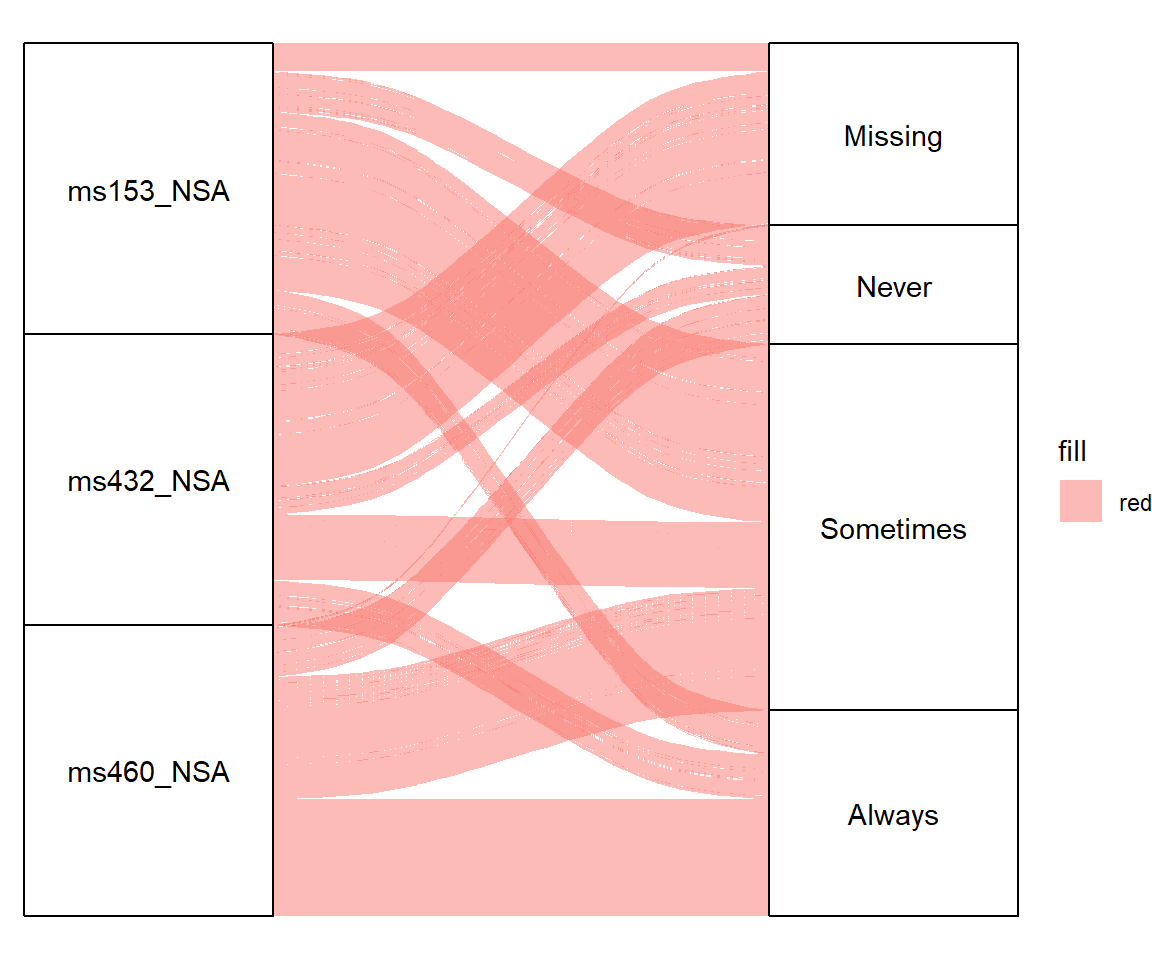Color palette

``````# install.packages("ggalluvial")
library(ggalluvial)

ggplot(data = vaccinations,
aes(axis1 = survey, axis2 = response, y = freq)) +
geom_alluvium(aes(fill = response)) +
geom_stratum() +
geom_text(stat = "stratum",
aes(label = after_stat(stratum))) +
scale_x_discrete(limits = c("Survey", "Response"),
expand = c(0.15, 0.05)) +
scale_fill_viridis_d() +
theme_void()``````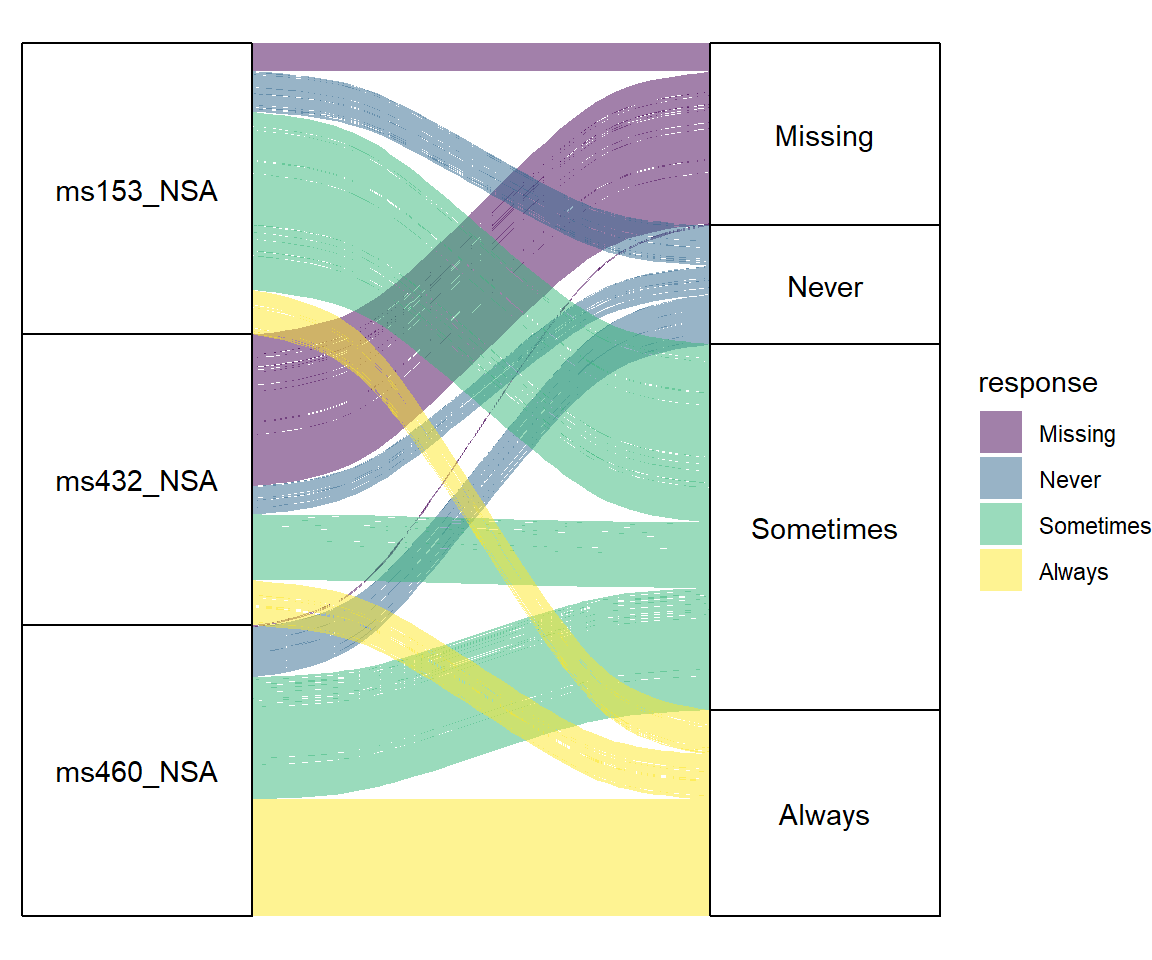Custom colors

``````# install.packages("ggalluvial")
library(ggalluvial)

# Colors
colors <- hcl.colors(4, "Red-Blue")

ggplot(data = vaccinations,
aes(axis1 = survey, axis2 = response, y = freq)) +
geom_alluvium(aes(fill = response)) +
geom_stratum() +
geom_text(stat = "stratum",
aes(label = after_stat(stratum))) +
scale_x_discrete(limits = c("Survey", "Response"),
expand = c(0.15, 0.05)) +
scale_fill_manual(values = colors) +
theme_void()``````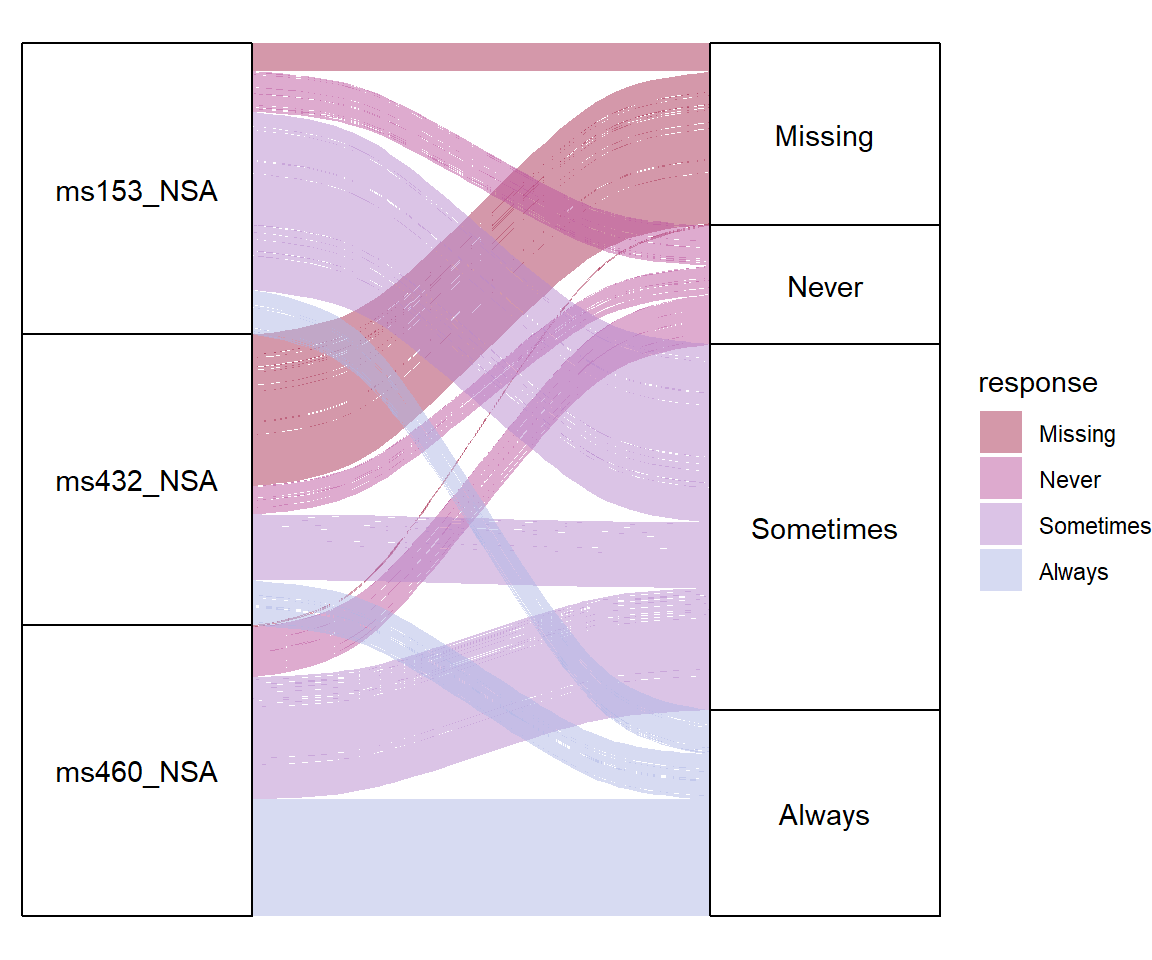Stratum color

``````# install.packages("ggalluvial")
library(ggalluvial)

ggplot(data = vaccinations,
aes(axis1 = survey, axis2 = response, y = freq)) +
geom_alluvium(aes(fill = response)) +
geom_stratum(aes(fill = response)) +
geom_text(stat = "stratum",
aes(label = after_stat(stratum))) +
scale_x_discrete(limits = c("Survey", "Response"),
expand = c(0.15, 0.05)) +
theme_void()``````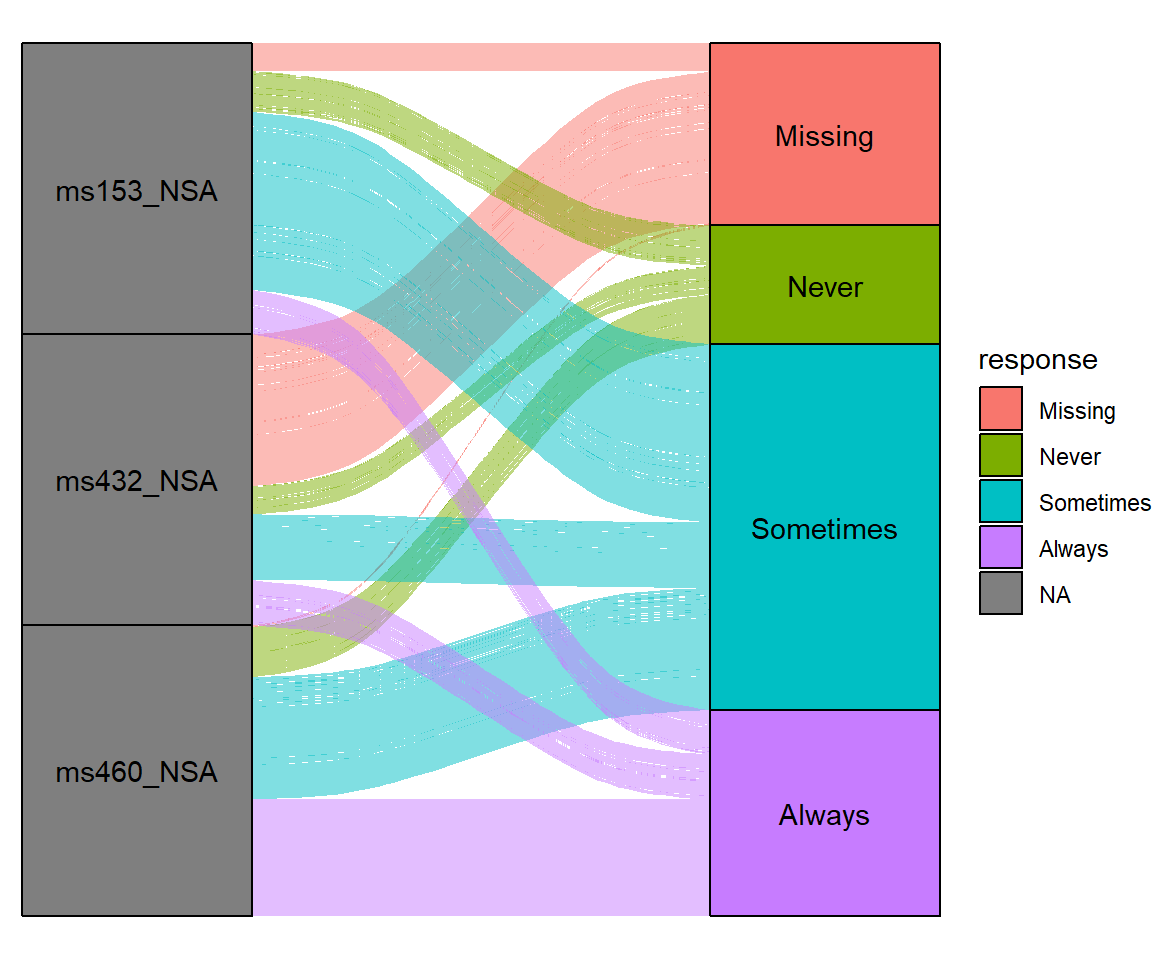## Legend customization

As the `ggalluvial` package was built with ggplot2 it is possible to customize the legend of the plots, such as changing its title, the key labels, its position or remove it as shown in the following examples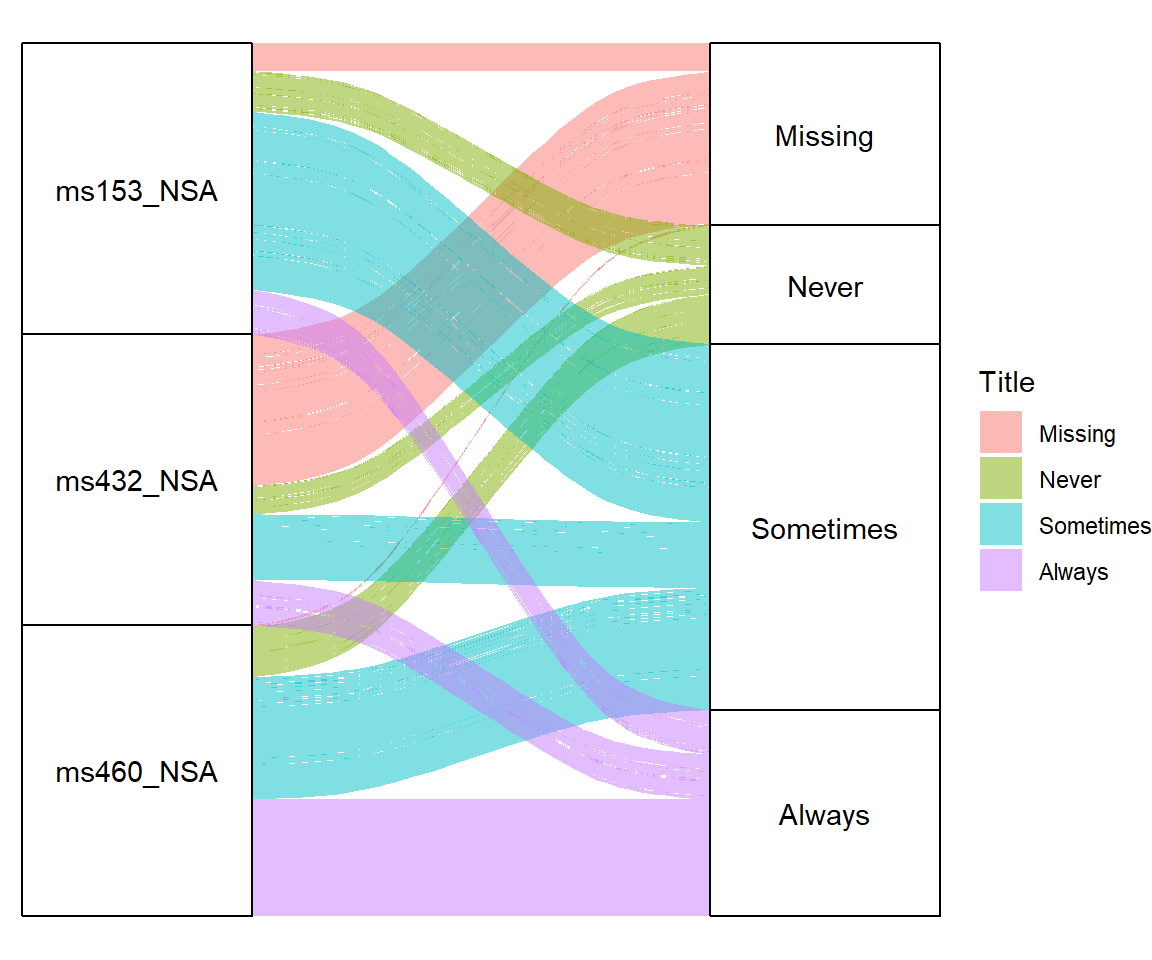Legend title

``````# install.packages("ggalluvial")
library(ggalluvial)

ggplot(data = vaccinations,
aes(axis1 = survey, axis2 = response, y = freq)) +
geom_alluvium(aes(fill = response)) +
geom_stratum() +
geom_text(stat = "stratum",
aes(label = after_stat(stratum))) +
scale_x_discrete(limits = c("Survey", "Response"),
expand = c(0.15, 0.05)) +
theme_void() +
guides(fill = guide_legend(title = "Title"))``````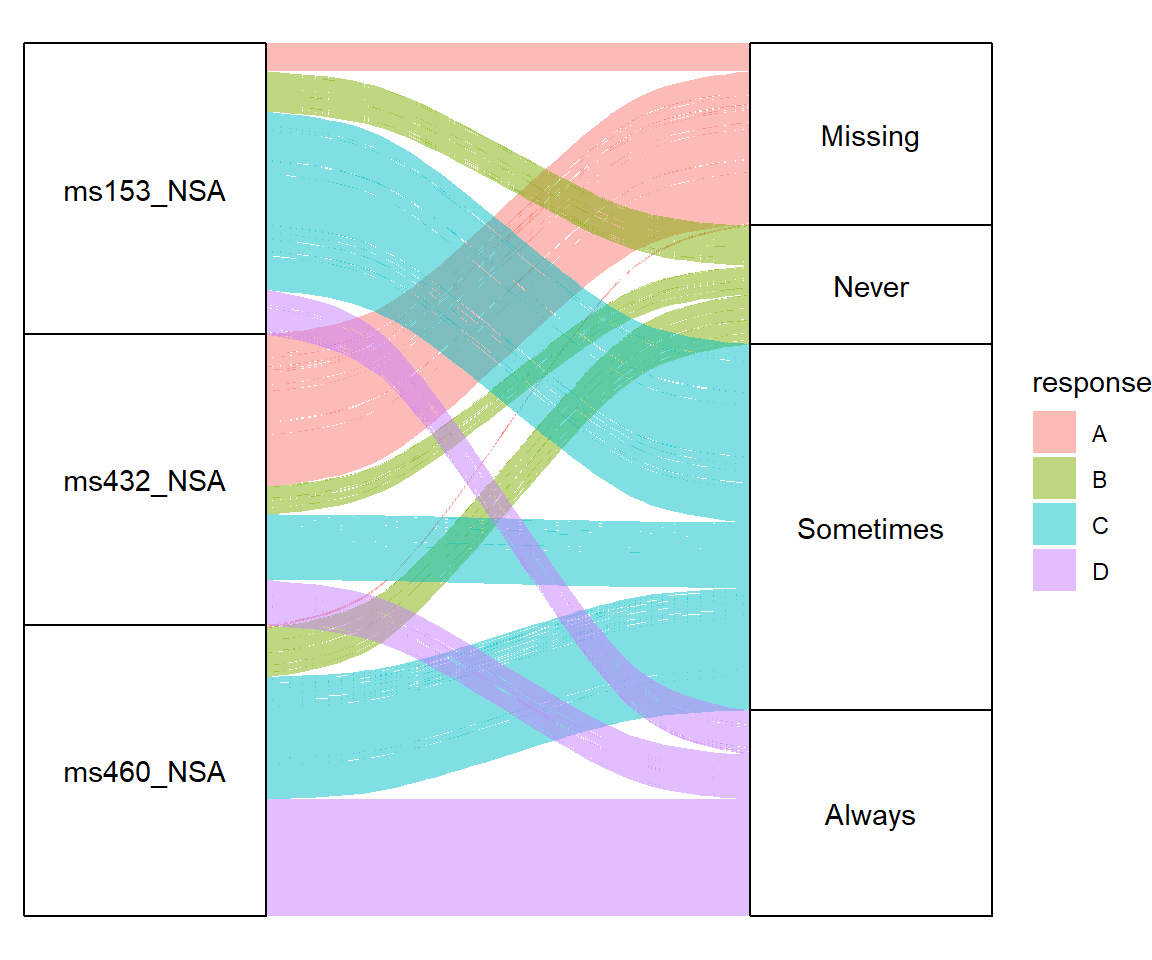Legend key labels

``````# install.packages("ggalluvial")
library(ggalluvial)

ggplot(data = vaccinations,
aes(axis1 = survey, axis2 = response, y = freq)) +
geom_alluvium(aes(fill = response)) +
geom_stratum() +
geom_text(stat = "stratum",
aes(label = after_stat(stratum))) +
scale_x_discrete(limits = c("Survey", "Response"),
expand = c(0.15, 0.05)) +
theme_void() +
scale_fill_hue(labels = c("A", "B", "C", "D"))``````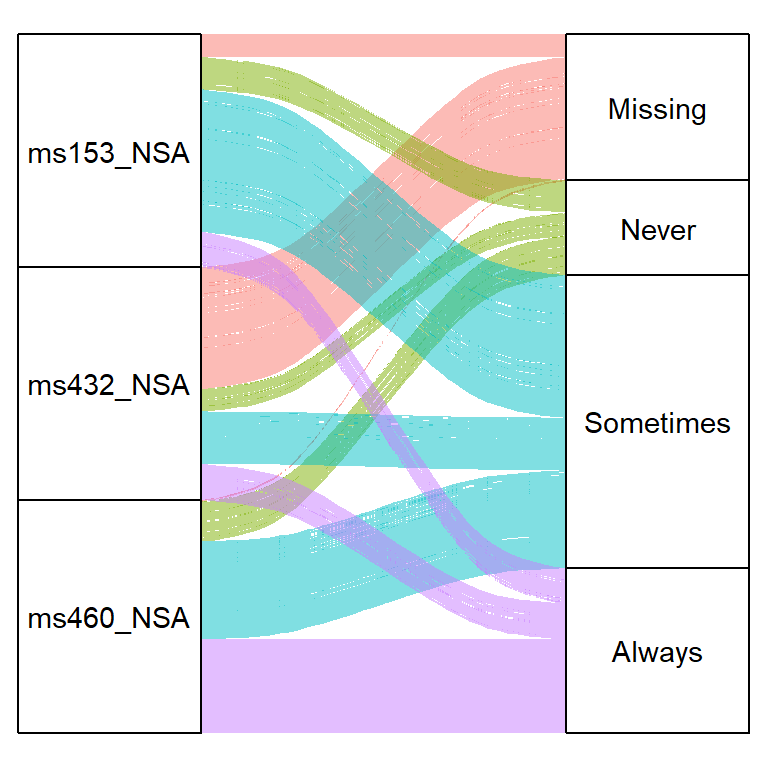Remove the legend

``````# install.packages("ggalluvial")
library(ggalluvial)

ggplot(data = vaccinations,
aes(axis1 = survey, axis2 = response, y = freq)) +
geom_alluvium(aes(fill = response)) +
geom_stratum() +
geom_text(stat = "stratum",
aes(label = after_stat(stratum))) +
scale_x_discrete(limits = c("Survey", "Response"),
expand = c(0.15, 0.05)) +
theme_void() +
theme(legend.position = "none")``````